## 6.4 Polarity of Molecules

### Learning Objectives

By the end of this section, you will be able to:

• Describe the formation of covalent bonds
• Define electronegativity and assess the polarity of covalent bonds

## Lewis Structures

We also use Lewis symbols to indicate the formation of covalent bonds, which are shown in Lewis structures, drawings that describe the bonding in molecules and polyatomic ions. For example, when two chlorine atoms form a chlorine molecule, they share one pair of electrons: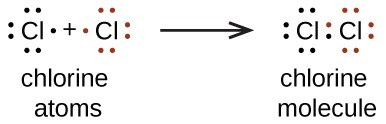The Lewis structure indicates that each Cl atom has three pairs of electrons that are not used in bonding (called lone pairs) and one shared pair of electrons (written between the atoms). A dash (or line) is sometimes used to indicate a shared pair of electrons:

A single shared pair of electrons is called a single bond. Each Cl atom interacts with eight valence electrons: the six in the lone pairs and the two in the single bond.

## Pure vs. Polar Covalent Bonds

If the atoms that form a covalent bond are identical, as in H2, Cl2, and other diatomic molecules, then the electrons in the bond must be shared equally. We refer to this as a pure covalent bond. Electrons shared in pure covalent bonds have an equal probability of being near each nucleus.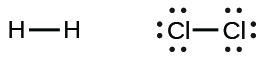In the case of Cl2, each atom starts off with seven valence electrons, and each Cl shares one electron with the other, forming one covalent bond:

$\text{Cl}+\text{Cl}\rightarrow{\text{Cl}}_{2}$

The total number of electrons around each individual atom consists of six nonbonding electrons and two shared (i.e., bonding) electrons for eight total electrons, matching the number of valence electrons in the noble gas argon. Since the bonding atoms are identical, Cl2 also features a pure covalent bond.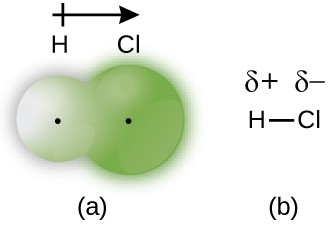Figure 2. (a) The distribution of electron density in the HCl molecule is uneven. The electron density is greater around the chlorine nucleus. The small, black dots indicate the location of the hydrogen and chlorine nuclei in the molecule. (b) Symbols δ+ and δ– indicate the polarity of the H–Cl bond.

When the atoms linked by a covalent bond are different, the bonding electrons are shared, but no longer equally. Instead, the bonding electrons are more attracted to one atom than the other, giving rise to a shift of electron density toward that atom. This unequal distribution of electrons is known as a polar covalent bond, characterized by a partial positive charge on one atom and a partial negative charge on the other. The atom that attracts the electrons more strongly acquires the partial negative charge and vice versa. For example, the electrons in the H–Cl bond of a hydrogen chloride molecule spend more time near the chlorine atom than near the hydrogen atom. Thus, in an HCl molecule, the chlorine atom carries a partial negative charge and the hydrogen atom has a partial positive charge. Figure 2 shows the distribution of electrons in the H–Cl bond. Note that the shaded area around Cl is much larger than it is around H. Compare this to Figure 1, which shows the even distribution of electrons in the H2 nonpolar bond.

We sometimes designate the positive and negative atoms in a polar covalent bond using a lowercase Greek letter “delta,” δ, with a plus sign or minus sign to indicate whether the atom has a partial positive charge (δ+) or a partial negative charge (δ–). This symbolism is shown for the H–Cl molecule in Figure 2.

## Electronegativity

Whether a bond is nonpolar or polar covalent is determined by a property of the bonding atoms called electronegativity. Electronegativity is a measure of the tendency of an atom to attract electrons (or electron density) towards itself. It determines how the shared electrons are distributed between the two atoms in a bond. The more strongly an atom attracts the electrons in its bonds, the larger its electronegativity. Electrons in a polar covalent bond are shifted toward the more electronegative atom; thus, the more electronegative atom is the one with the partial negative charge. The greater the difference in electronegativity, the more polarized the electron distribution and the larger the partial charges of the atoms.

Figure 3 shows the electronegativity values of the elements as proposed by one of the most famous chemists of the twentieth century: Linus Pauling (Figure 4). In general, electronegativity increases from left to right across a period in the periodic table and decreases down a group. Thus, the nonmetals, which lie in the upper right, tend to have the highest electronegativities, with fluorine the most electronegative element of all (EN = 4.0). Metals tend to be less electronegative elements, and the group 1 metals have the lowest electronegativities. Note that noble gases are excluded from this figure because these atoms usually do not share electrons with others atoms since they have a full valence shell. (While noble gas compounds such as XeO2 do exist, they can only be formed under extreme conditions, and thus they do not fit neatly into the general model of electronegativity.)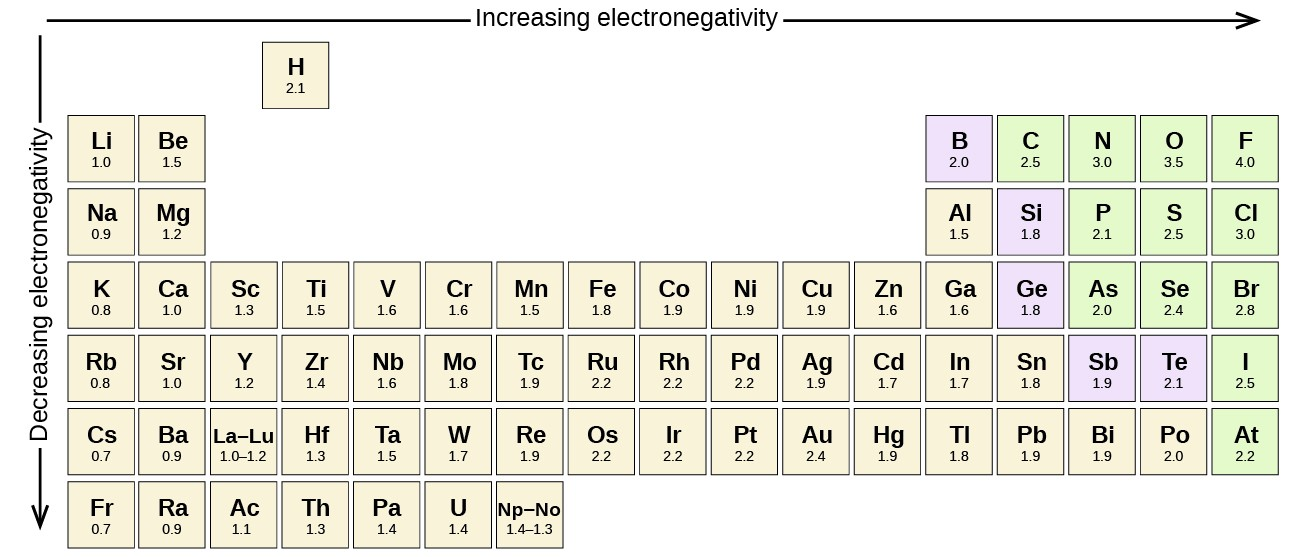Figure 3. The electronegativity values derived by Pauling follow predictable periodic trends with the higher electronegativities toward the upper right of the periodic table.

### Portrait of a Chemist: Linus Pauling

Linus Pauling, shown in Figure 4, is the only person to have received two unshared (individual) Nobel Prizes: one for chemistry in 1954 for his work on the nature of chemical bonds and one for peace in 1962 for his opposition to weapons of mass destruction. He developed many of the theories and concepts that are foundational to our current understanding of chemistry, including electronegativity and resonance structures.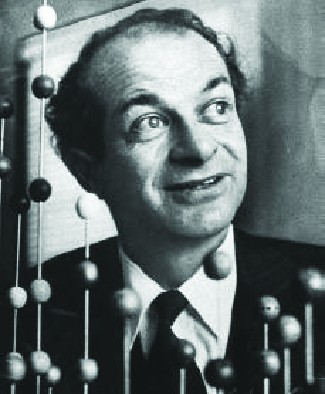Figure 4. Linus Pauling (1901–1994) made many important contributions to the field of chemistry. He was also a prominent activist, publicizing issues related to health and nuclear weapons.

Pauling also contributed to many other fields besides chemistry. His research on sickle cell anemia revealed the cause of the disease—the presence of a genetically inherited abnormal protein in the blood—and paved the way for the field of molecular genetics. His work was also pivotal in curbing the testing of nuclear weapons; he proved that radioactive fallout from nuclear testing posed a public health risk.

### Electronegativity and Bond Type

The absolute value of the difference in electronegativity (ΔEN) of two bonded atoms provides a rough measure of the polarity to be expected in the bond and, thus, the bond type. When the difference is very small or zero, the bond is covalent and nonpolar. When it is large, the bond is polar covalent or ionic. The absolute values of the electronegativity differences between the atoms in the bonds H–H, H–Cl, and Na–Cl are 0 (nonpolar), 0.9 (polar covalent), and 2.1 (ionic), respectively. The degree to which electrons are shared between atoms varies from completely equal (pure covalent bonding) to not at all (ionic bonding). Figure 5 shows the relationship between electronegativity difference and bond type.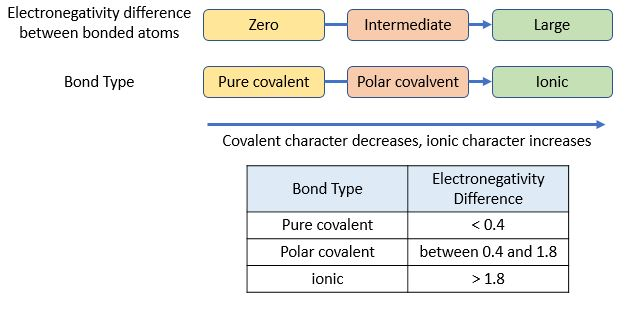Figure 5. As the electronegativity difference increases between two atoms, the bond becomes more ionic.

A rough approximation of the electronegativity differences associated with covalent, polar covalent, and ionic bonds is shown in Figure 5. This table is just a general guide, however, with many exceptions. For example, the H and F atoms in HF have an electronegativity difference of 1.9, and the N and H atoms in NH3 a difference of 0.9, yet both of these compounds form bonds that are considered polar covalent. Likewise, the Na and Cl atoms in NaCl have an electronegativity difference of 2.1, and the Mn and I atoms in MnI2 have a difference of 1.0, yet both of these substances form ionic compounds.

The best guide to the covalent or ionic character of a bond is to consider the types of atoms involved and their relative positions in the periodic table. Bonds between two nonmetals are generally covalent; bonding between a metal and a nonmetal is often ionic.

Some compounds contain both covalent and ionic bonds. The atoms in polyatomic ions, such as OH, ${\text{NO}}_{3}^{-},$ and ${\text{NH}}_{4}^{\text{+}},$ are held together by polar covalent bonds. However, these polyatomic ions form ionic compounds by combining with ions of opposite charge. For example, potassium nitrate, KNO3, contains the K+ cation and the polyatomic ${\text{NO}}_{3}^{-}$ anion. Thus, bonding in potassium nitrate is ionic, resulting from the electrostatic attraction between the ions K+ and ${\text{NO}}_{3}^{-},$ as well as covalent between the nitrogen and oxygen atoms in ${\text{NO}}_{3}^{-}$.

### Example 1: Electronegativity and Bond Polarity

Bond polarities play an important role in determining the structure of proteins. Using the electronegativity values in Figure 7.6, arrange the following covalent bonds—all commonly found in amino acids—in order of increasing polarity. Then designate the positive and negative atoms using the symbols δ+ and δ–:

C–H, C–N, C–O, N–H, O–H, S–H

Silicones are polymeric compounds containing, among others, the following types of covalent bonds: Si–O, Si–C, C–H, and C–C. Using the electronegativity values in Figure 3, arrange the bonds in order of increasing polarity and designate the positive and negative atoms using the symbols δ+ and δ–.

## Molecular Polarity and Dipole Moment

As discussed previously, polar covalent bonds connect two atoms with differing electronegativities, leaving one atom with a partial positive charge (Δ+) and the other atom with a partial negative charge (Δ–), as the electrons are pulled toward the more electronegative atom. This separation of charge gives rise to a bond dipole moment. The magnitude of a bond dipole moment is represented by the Greek letter mu (µ) and is given by the formula shown below, where Q is the magnitude of the partial charges (determined by the electronegativity difference) and r is the distance between the charges:

$\mu =\text{Qr}$

This bond moment can be represented as a vector, a quantity having both direction and magnitude (Figure 13). Dipole vectors are shown as arrows pointing along the bond from the less electronegative atom toward the more electronegative atom. A small plus sign is drawn on the less electronegative end to indicate the partially positive end of the bond. The length of the arrow is proportional to the magnitude of the electronegativity difference between the two atoms.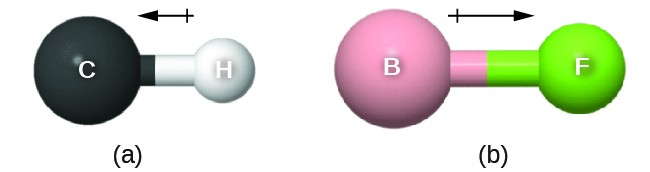Figure 13. (a) There is a small difference in electronegativity between C and H, represented as a short vector. (b) The electronegativity difference between B and F is much larger, so the vector representing the bond moment is much longer.

A whole molecule may also have a separation of charge, depending on its molecular structure and the polarity of each of its bonds. If such a charge separation exists, the molecule is said to be a polar molecule (or dipole); otherwise the molecule is said to be nonpolar. The dipole moment measures the extent of net charge separation in the molecule as a whole. We determine the dipole moment by adding the bond moments in three-dimensional space, taking into account the molecular structure.

For diatomic molecules, there is only one bond, so its bond dipole moment determines the molecular polarity. Homonuclear diatomic molecules such as Br2 and N2 have no difference in electronegativity, so their dipole moment is zero. For heteronuclear molecules such as CO, there is a small dipole moment. For HF, there is a larger dipole moment because there is a larger difference in electronegativity.

When a molecule contains more than one bond, the geometry must be taken into account. If the bonds in a molecule are arranged such that their bond moments cancel (vector sum equals zero), then the molecule is nonpolar. This is the situation in CO2 (Figure 14). Each of the bonds is polar, but the molecule as a whole is nonpolar. From the Lewis structure, and using VSEPR theory, we determine that the CO2 molecule is linear with polar C=O bonds on opposite sides of the carbon atom. The bond moments cancel because they are pointed in opposite directions. In the case of the water molecule (Figure 14), the Lewis structure again shows that there are two bonds to a central atom, and the electronegativity difference again shows that each of these bonds has a nonzero bond moment. In this case, however, the molecular structure is bent because of the lone pairs on O, and the two bond moments do not cancel. Therefore, water does have a net dipole moment and is a polar molecule (dipole).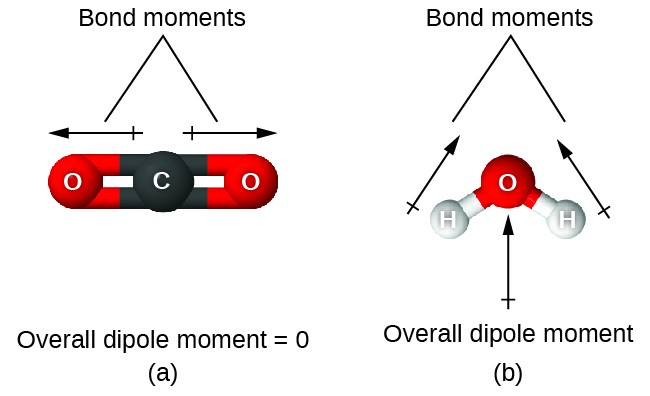Figure 14. The overall dipole moment of a molecule depends on the individual bond dipole moments and how they are arranged. (a) Each CO bond has a bond dipole moment, but they point in opposite directions so that the net CO2 molecule is nonpolar. (b) In contrast, water is polar because the OH bond moments do not cancel out.

The OCS molecule has a structure similar to CO2, but a sulfur atom has replaced one of the oxygen atoms. To determine if this molecule is polar, we draw the molecular structure. VSEPR theory predicts a linear molecule: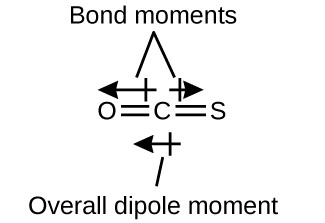Although the C–O bond is polar, C and S have the same electronegativity values as shown in Figure 15, so there is no C–S dipole. Thus, the two bonds do not have of the same bond dipole moment, and the bond moments do not cancel. Because oxygen is more electronegative than sulfur, the oxygen end of the molecule is the negative end.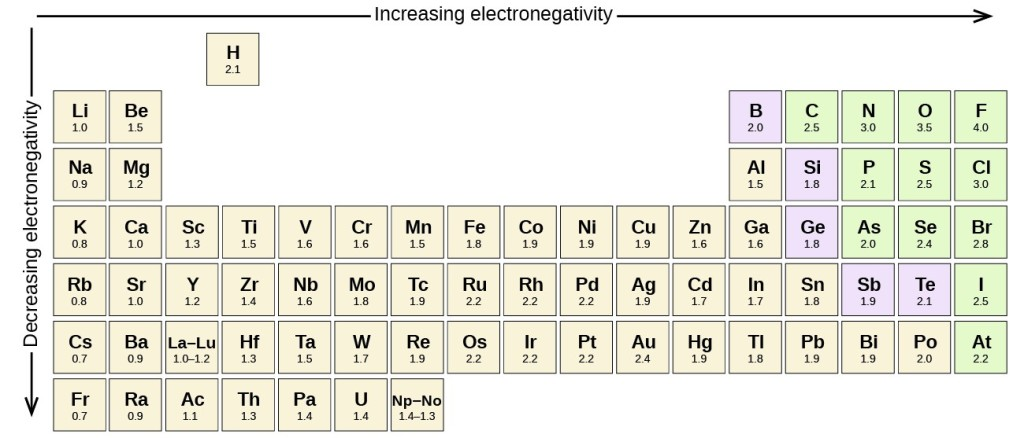Figure 15. The electronegativity values derived by Pauling follow predictable periodic trends with the higher electronegativities toward the upper right of the periodic table.

Chloromethane, CH3Cl, is another example of a polar molecule. Although the polar C–Cl and C–H bonds are arranged in a tetrahedral geometry, the C–Cl bonds have a larger bond moment than the C–H bond, and the bond moments do not completely cancel each other. All of the dipoles have a upward component in the orientation shown, since carbon is more electronegative than hydrogen and less electronegative than chlorine:

When we examine the highly symmetrical molecules BF3 (trigonal planar), CH4 (tetrahedral), PF5 (trigonal bipyramidal), and SF6 (octahedral), in which all the polar bonds are identical, the molecules are nonpolar. The bonds in these molecules are arranged such that their dipoles cancel. However, just because a molecule contains identical bonds does not mean that the dipoles will always cancel. Many molecules that have identical bonds and lone pairs on the central atoms have bond dipoles that do not cancel. Examples include H2S and NH3. A hydrogen atom is at the positive end and a nitrogen or sulfur atom is at the negative end of the polar bonds in these molecules:To summarize, to be polar, a molecule must:

1. Contain at least one polar covalent bond.
2. Have a molecular structure such that the sum of the vectors of each bond dipole moment does not cancel.

## Properties of Polar Molecules

Polar molecules tend to align when placed in an electric field with the positive end of the molecule oriented toward the negative plate and the negative end toward the positive plate (Figure 16). We can use an electrically charged object to attract polar molecules, but nonpolar molecules are not attracted. Also, polar solvents are better at dissolving polar substances, and nonpolar solvents are better at dissolving nonpolar substances.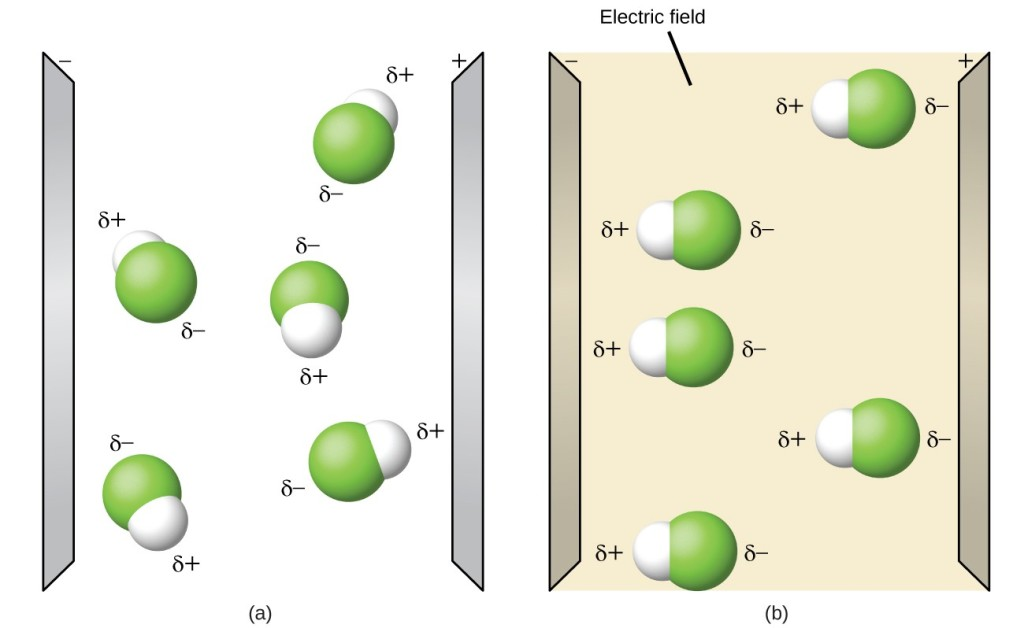Figure 16. (a) Molecules are always randomly distributed in the liquid state in the absence of an electric field. (b) When an electric field is applied, polar molecules like HF will align to the dipoles with the field direction.

The PhET molecule polarity simulation provides many ways to explore dipole moments of bonds and molecules.

### Example 2: Polarity Simulations

Open the PhET molecule polarity simulation and select the “Three Atoms” tab at the top. This should display a molecule ABC with three electronegativity adjustors. You can display or hide the bond moments, molecular dipoles, and partial charges at the right. Turning on the Electric Field will show whether the molecule moves when exposed to a field, similar to Figure 15.

Use the electronegativity controls to determine how the molecular dipole will look for the starting bent molecule if:

1. A and C are very electronegative and B is in the middle of the range.
2. A is very electronegative, and B and C are not.

Determine the partial charges that will give the largest possible bond dipoles.

### Key Concepts and Summary

A dipole moment measures a separation of charge. For one bond, the bond dipole moment is determined by the difference in electronegativity between the two atoms. For a molecule, the overall dipole moment is determined by both the individual bond moments and how these dipoles are arranged in the molecular structure. Polar molecules (those with an appreciable dipole moment) interact with electric fields, whereas nonpolar molecules do not.

### Exercises

1. Explain how a molecule that contains polar bonds can be nonpolar.
2. Which of the following molecules contain polar bonds? Which of these molecules and ions have dipole moments?
1. PCl3
2. CS2
3. CCl2F2
4. ClNO (N is the central atom)
5. CF4
6. CH3Cl
7. H2CO
3. Use the PhET simulation to perform the following exercises for a two-atom molecule:
1. Adjust the electronegativity value so the bond dipole is pointing toward B. Then determine what the electronegativity values must be to switch the dipole so that it points toward A.
2. With a partial positive charge on A, turn on the electric field and describe what happens.
3. With a small partial negative charge on A, turn on the electric field and describe what happens.
4. Reset all, and then with a large partial negative charge on A, turn on the electric field and describe what happens.
4. Use the PhET simulation to perform the following exercises for a real molecule. You may need to rotate the molecules in three dimensions to see certain dipoles.
1. Look at the bond dipoles for NH3. Use these dipoles to predict whether N or H is more electronegative.
2. Predict whether there should be a molecular dipole for NH3 and, if so, in which direction it will point. Check the molecular dipole box to test your hypothesis.

### Key Concepts and Summary

Covalent bonds form when electrons are shared between atoms and are attracted by the nuclei of both atoms. In pure covalent bonds, the electrons are shared equally. In polar covalent bonds, the electrons are shared unequally, as one atom exerts a stronger force of attraction on the electrons than the other. The ability of an atom to attract a pair of electrons in a chemical bond is called its electronegativity. The difference in electronegativity between two atoms determines how polar a bond will be. In a diatomic molecule with two identical atoms, there is no difference in electronegativity, so the bond is nonpolar or pure covalent. When the electronegativity difference is very large, as is the case between metals and nonmetals, the bonding is characterized as ionic.

## Glossary

bond dipole moment: separation of charge in a bond that depends on the difference in electronegativity and the bond distance represented by partial charges or a vector

bond length: distance between the nuclei of two bonded atoms at which the lowest potential energy is achieved

covalent bond: bond formed when electrons are shared between atoms

dipole moment: property of a molecule that describes the separation of charge determined by the sum of the individual bond moments based on the molecular structure

electronegativity: tendency of an atom to attract electrons in a bond to itself

polar covalent bond: covalent bond between atoms of different electronegativities; a covalent bond with a positive end and a negative end

polar molecule: (also, dipole) molecule with an overall dipole moment

pure covalent bond: (also, nonpolar covalent bond) covalent bond between atoms of identical electronegativities